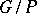# Schubert cycle

(diff) ← Older revision | Latest revision (diff) | Newer revision → (diff)
The cycle class of a Schubert variety in the cohomology ring of a complex flag manifold(cf. also Flag structure), also called a Schubert class. Here,is a semi-simple linear algebraic group andis a parabolic subgroup. Schubert cycles form a basis for the cohomology groups [a4], [a1], 14.12, of(cf. also Cohomology group). They arose [a4] as representatives of Schubert conditions on linear subspaces of a vector space in the Schubert calculus for enumerative geometry [a3]. The justification of Schubert's calculus in this context by C. Ehresmann [a2] realized Schubert cycles as cohomology classes Poincaré dual to the fundamental homology cycles of Schubert varieties in the Grassmannian. While Schubert, Ehresmann and others worked primarily on the Grassmannian, the pertinent features of the Grassmannian extend to general flag varieties, giving Schubert cycles as above.
More generally, whenis a semi-simple linear algebraic group over a field, there are Schubert cycles associated to Schubert varieties in each of the following theories for: singular (or de Rham) cohomology, the Chow ring, K-theory, or equivariant or quantum versions of these theories. For each, the Schubert cycles form a basis over the base ring. For the cohomology or the Chow ring, the Schubert cycles are universal characteristic classes for (flagged)-bundles. In particular, certain special Schubert cycles for the Grassmannian are universal Chern classes for vector bundles (cf. also Chern class).